# Cylindrical Function

## cylindrical function

[sə′lin·dri·kəl ‚fəŋk·shən]
(mathematics)
McGraw-Hill Dictionary of Scientific & Technical Terms, 6E, Copyright © 2003 by The McGraw-Hill Companies, Inc.
The following article is from The Great Soviet Encyclopedia (1979). It might be outdated or ideologically biased.

## Cylindrical Function

(or cylinder function, Bessel function). Cylindrical functions form a class of transcendental functions that is extremely important from the standpoint of applications in physics and engineering. These transcendental functions are solutions of the differential equation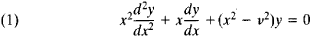where v is an arbitrary parameter. Many problems of elastic, thermal, and electrical equilibrium and of the vibration of bodies of cylindrical shape reduce to this equation.

A solution of the form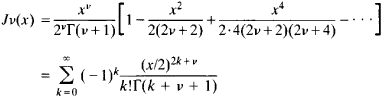is called a cylindrical function of order v of the first kind. Here, Γ(z) is the gamma function; the series on the right-hand side of the equation converges for all values of x. In particular, the zeroth-order cylindrical function has the form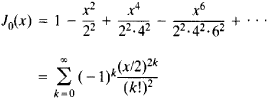If v is a negative integer (v = – n), then Jv(x) is defined in the following way: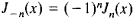Cylindrical functions of order v = m + 1/2, where m is an integer, reduce to elementary functions—for example,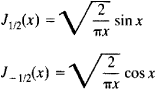The functions Jv(x) are often called simply Bessel functions, and equation (1) is known as the Bessel equation. These functions and equation (1), however, were used by L. Euler in a study of the vibrations of a membrane in 1766—that is, nearly 50 years before the work of F. Bessel. The zeroth-order function occurred still earlier in a paper by D. Bernoulli devoted to the oscillation of a heavy chain (published 1738), and the function of order 1/3 is found in a letter from Jakob Bernoulli to G. von Leibnitz (1703).

If v is not an integer, then the general solution of equation (1) has the form

(2) y = C1Jv(x) + C2Jv(x)

where C1 and C2 are constants. If, however, v is an integer, then Jv(x) and Jv(x) are linearly dependent, and their linear combination (2) is not a general solution of equation (1). For this reason, cylindrical functions of the second kind, or Neumann functions, were introduced:With the aid of these functions the general solution of equation (1) may be written in the form

y = C1Jv(x) + C2Jv(x)

for both integral and nonintegral v.

In applications cylindrical functions of an imaginary argument are encountered:(the modified cylindrical function of the first kind) and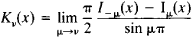(the MacDonald function, or modified cylindrical function of the second kind). These functions satisfy the equation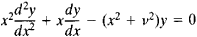the general solution of which has the form

y = C1Iv(x) + C2Kv(x

for both integral and nonintegral v.

Cylindrical functions of the third kind, or Hankel functions, are frequently used: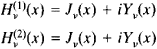The functions ber (x) and bei (x) are also often encountered. They are defined by the equationAn important role is played by asymptotic expressions of cylindrical functions for large values of the argument: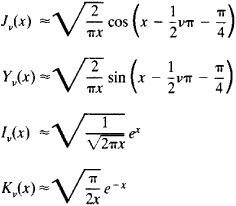It follows from these expressions in particular that the cylindrical functions Jv(x) and Yv(x) have an infinite set of real zeros arranged in such a way that far from the origin they are arbitrarily close to the zeros of the functions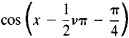and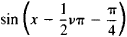respectively.

Cylindrical functions have been studied in great detail for complex values of the arguments. Many tables of cylindrical functions have been published for use in calculations.

### REFERENCES

Smirnov, V. I. Kurs vysshei matematiki, 8th ed., vol. 3, part 2. Moscow, 1969.
Nikiforov, A. F., and V. B. Uvarov. Osnovy teorii spetsial’nykh funktsii. Moscow, 1974.
Watson, G. N. Teoriia besselevykh funktsii, parts 1–2. Moscow, 1949. (Translated from English.)
Erdelyi, A., et al., eds. Vysshie transtsendentnye funktsii, 2nd ed., vol. 2. Moscow, 1974. (Translated from English.)
The Great Soviet Encyclopedia, 3rd Edition (1970-1979). © 2010 The Gale Group, Inc. All rights reserved.
References in periodicals archive ?
Let f be a cylindrical function associated with the finite set of indexes [??] = {[[lambda].sub.1], ..., [[lambda].sub.n]} and with the function [mathematical expression not reproducible].
The mean value of a cylindrical function f is immediately computed, since its trace is defined on [[PI].sub.[lambda][member of][??]] [U.sub.[lambda],n] up to a subset of measure 0.
This shows that O[GAMMA] is an admissible domain in [X.sup.N] and enables us to write, for a bounded cylindrical function f,
For instance, the map [[phi].sub.s] defined in the example of Section 3.7 extends to a cylindrical function on [L.sup.2]-forms, and hence the construction described above applies to this extension map.
where [a.bar] is waves coupling coefficient, which indicates the proportion mixes E and H type waves in hybrid modes; [[A.bar].sub.1], [[B.bar].sub.1]--unknown amplitude coefficients; [J.sub.m]([[k.bar].sup.g.sub.[perpendicular to].sub.1,2]r) is the Bessel cylindrical function of the first kind m-th order; [[k.bar].sup.g.sub.[perpendicular to].sub.1,2]r - first and second internal transverse waves numbers normalized to radius r; m = 0, 1, 2, ...
where [J.sub.m]([k.sup.d.sub.[perpendicular to]r]) is the first kind Bessel and m-th order Neumann cylindrical functions with argument [k.sup.d.sub.[perpendicular to]r].
For calculation of cylindrical functions of complex argument, the AMOS Fortran library (http://netlib.org/amos/) was ported to C++.
First, a large computation time is required as the spectral Green's function components involve cylindrical functions. To speed up the calculations, we adopt the remedy applied during the evaluation of the input impedance of a CML-fed CMA (strong coupling) introduced in .
For the calculations of the presented examples the number of space harmonics L = 4, P = 6 with Q = 2 (for metallic structures) or Q = 6 (for dielectric structures) and number of cylindrical functions M = 7 were chosen which was sufficient to obtain convergence of the solution.
for f [left arrow], [DELTA]f, [f.sub.max] do 1) Evaluation of the coefficients : [MATHEMATICAL EXPRESSION NOT REPRODUCIBLE IN ASCII] 2) Evaluation of the cylindrical functions: [MATHEMATICAL EXPRESSION NOT REPRODUCIBLE IN ASCII] 3) Calculation of the determinant elements: [[bar.a].sub.jk] with j = 0, 1, ..., 8; and k = 0, 1, 8.
Furthermore, in order to avoid possible overflow/underflow problems in the numerical calculations of special cylindrical functions such as Bessel and Hankel functions, which arise in the MPGFs, a novel form of the spectral-domain MPGFs is developed.
Since in (13), all Bessel and Hankel functions are in the form of ratios, the possible overflow/underflow problems in the numerical calculations of special cylindrical functions in (15) can be avoided, and the efficiency can be improved.

Site: Follow: Share:
Open / Close